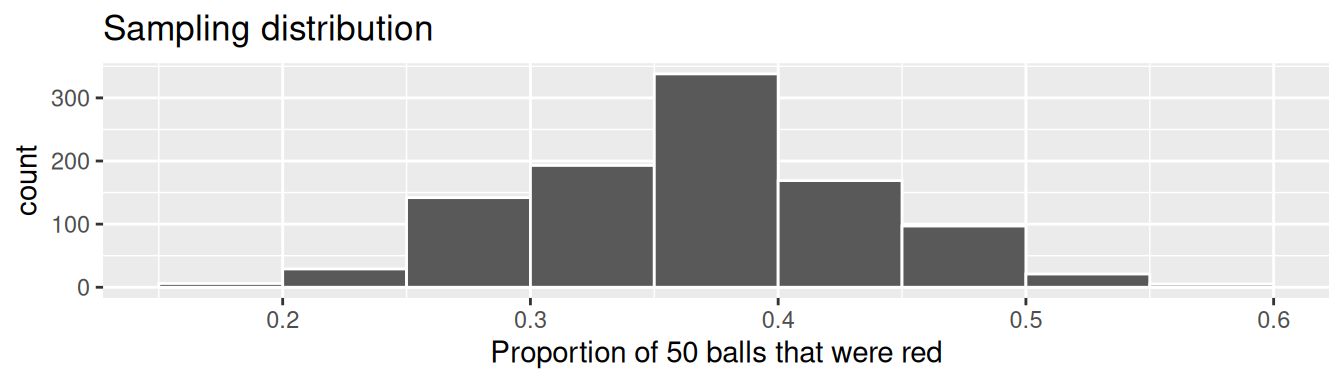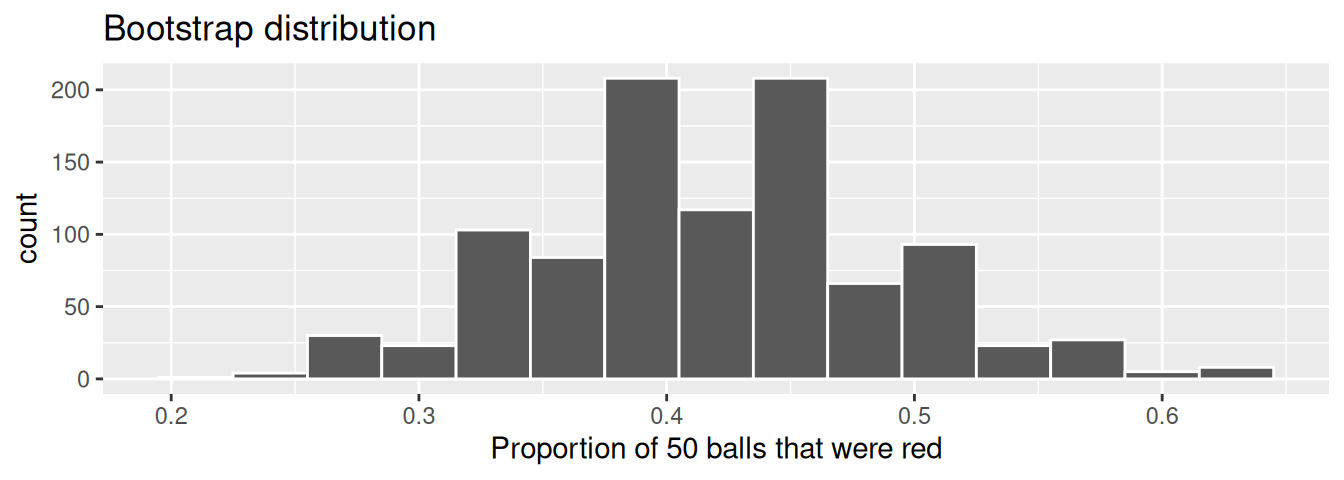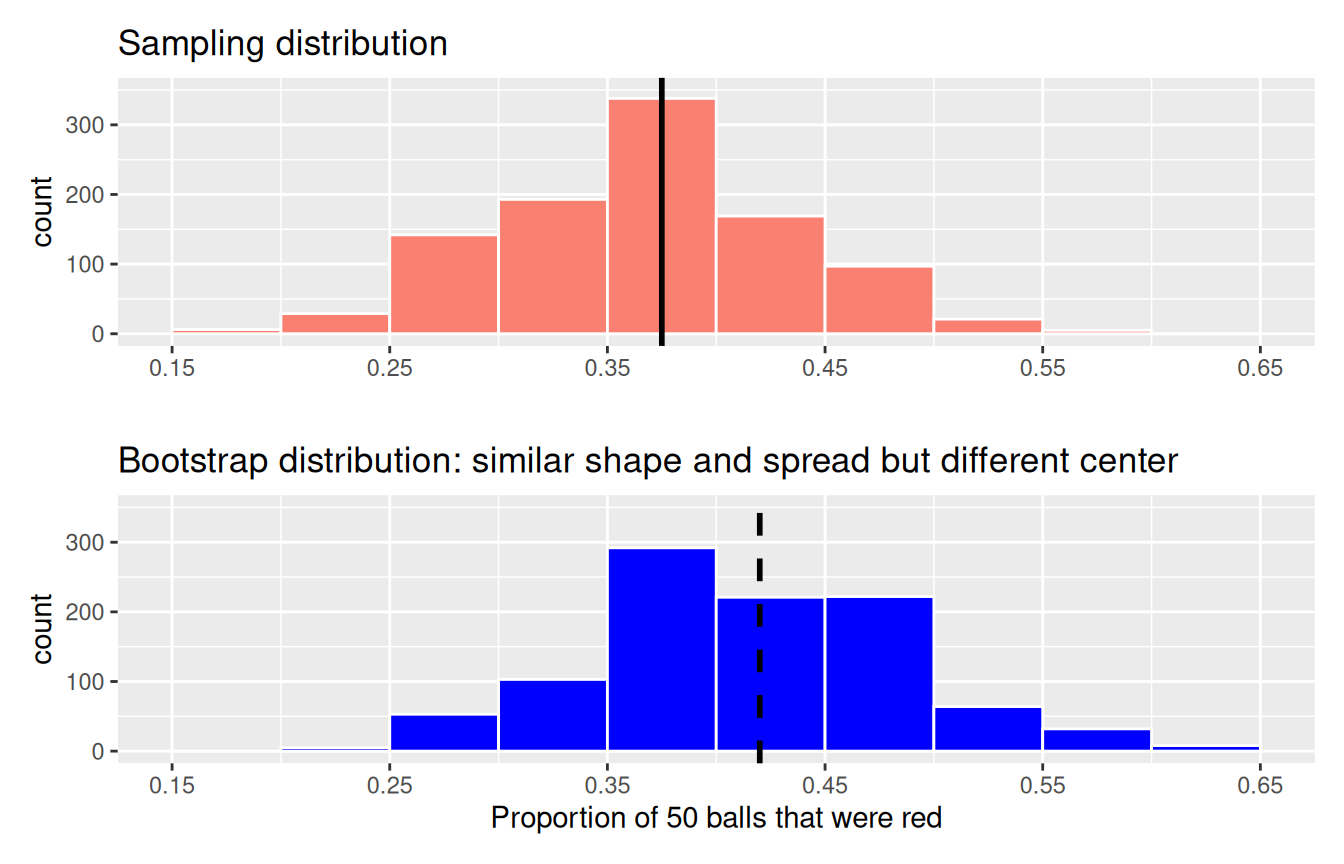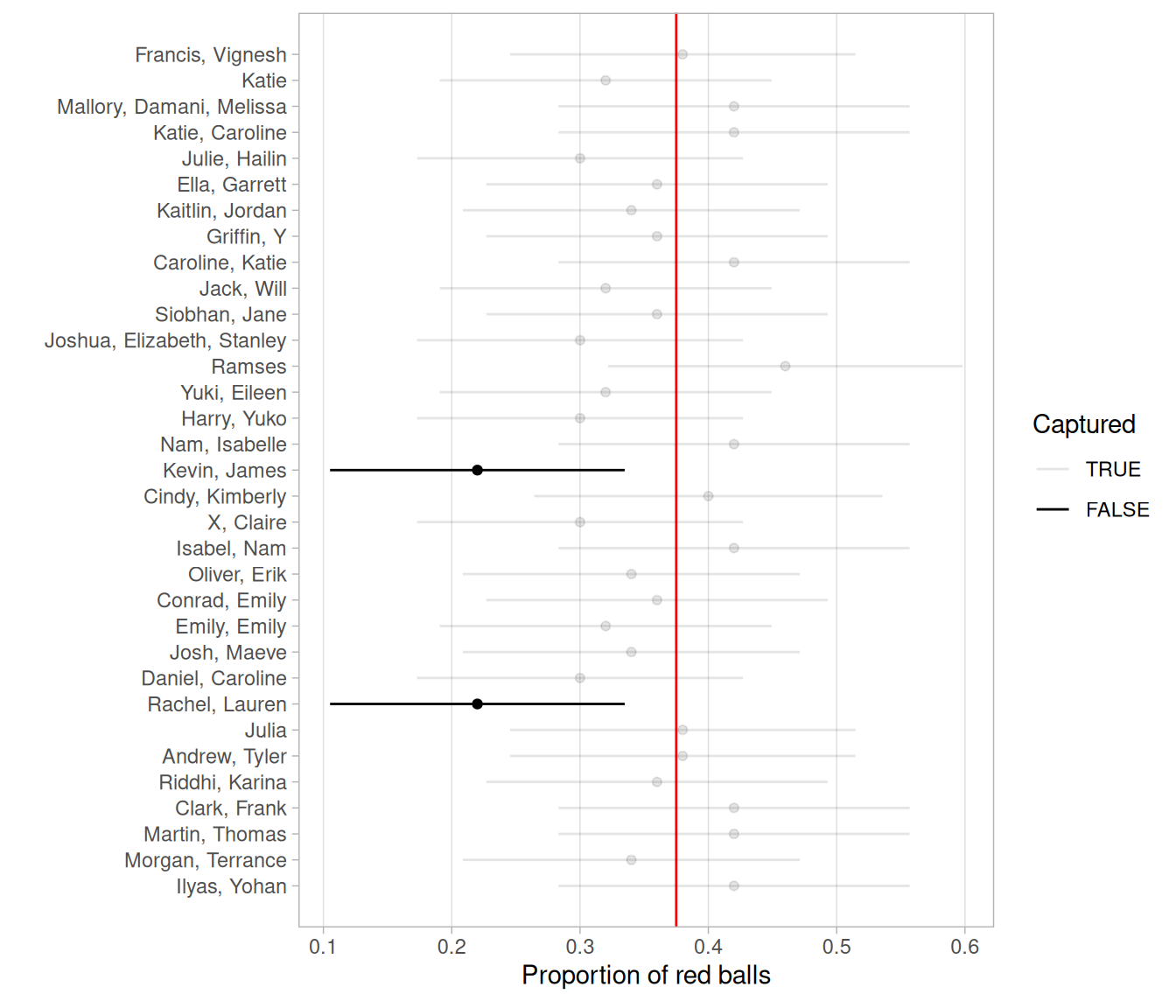## 8.7 Conclusion

### 8.7.1 Comparing bootstrap and sampling distributions

Let’s talk more about the relationship between sampling distributions and bootstrap distributions.

Recall back in Subsection 7.2.3, we took 1000 virtual samples from the bowl using a virtual shovel, computed 1000 values of the sample proportion red $$\widehat{p}$$, then visualized their distribution in a histogram. Recall that this distribution is called the sampling distribution of $$\widehat{p}$$. Furthermore, the standard deviation of the sampling distribution has a special name: the standard error.

We also mentioned that this sampling activity does not reflect how sampling is done in real life. Rather, it was an idealized version of sampling so that we could study the effects of sampling variation on estimates, like the proportion of the shovel’s balls that are red. In real life, however, one would take a single sample that’s as large as possible, much like in the Obama poll we saw in Section 7.4. But how can we get a sense of the effect of sampling variation on estimates if we only have one sample and thus only one estimate? Don’t we need many samples and hence many estimates?

The workaround to having a single sample was to perform bootstrap resampling with replacement from the single sample. We did this in the resampling activity in Section 8.1 where we focused on the mean year of minting of pennies. We used pieces of paper representing the original sample of 50 pennies from the bank and resampled them with replacement from a hat. We had 35 of our friends perform this activity and visualized the resulting 35 sample means $$\overline{x}$$ in a histogram in Figure 8.11.

This distribution was called the bootstrap distribution of $$\overline{x}$$. We stated at the time that the bootstrap distribution is an approximation to the sampling distribution of $$\overline{x}$$ in the sense that both distributions will have a similar shape and similar spread. Thus the standard error of the bootstrap distribution can be used as an approximation to the standard error of the sampling distribution.

Let’s show you that this is the case by now comparing these two types of distributions. Specifically, we’ll compare

1. the sampling distribution of $$\widehat{p}$$ based on 1000 virtual samples from the bowl from Subsection 7.2.3 to
2. the bootstrap distribution of $$\widehat{p}$$ based on 1000 virtual resamples with replacement from Ilyas and Yohan’s single sample bowl_sample_1 from Subsection 8.5.1.

#### Sampling distribution

Here is the code you saw in Subsection 7.2.3 to construct the sampling distribution of $$\widehat{p}$$ shown again in Figure 8.33, with some changes to incorporate the statistical terminology relating to sampling from Subsection 7.3.1.

# Take 1000 virtual samples of size 50 from the bowl:
virtual_samples <- bowl %>%
rep_sample_n(size = 50, reps = 1000)
# Compute the sampling distribution of 1000 values of p-hat
sampling_distribution <- virtual_samples %>%
group_by(replicate) %>%
summarize(red = sum(color == "red")) %>%
mutate(prop_red = red / 50)
# Visualize sampling distribution of p-hat
ggplot(sampling_distribution, aes(x = prop_red)) +
geom_histogram(binwidth = 0.05, boundary = 0.4, color = "white") +
labs(x = "Proportion of 50 balls that were red",
title = "Sampling distribution")FIGURE 8.33: Previously seen sampling distribution of sample proportion red for $$n = 1000$$.

An important thing to keep in mind is the default value for replace is FALSE when using rep_sample_n(). This is because when sampling 50 balls with a shovel, we are extracting 50 balls one-by-one without replacing them. This is in contrast to bootstrap resampling with replacement, where we resample a ball and put it back, and repeat this process 50 times.

Let’s quantify the variability in this sampling distribution by calculating the standard deviation of the prop_red variable representing 1000 values of the sample proportion $$\widehat{p}$$. Remember that the standard deviation of the sampling distribution is the standard error, frequently denoted as se.

sampling_distribution %>% summarize(se = sd(prop_red))
# A tibble: 1 x 1
se
<dbl>
1 0.0673987

#### Bootstrap distribution

Here is the code you previously saw in Subsection 8.5.1 to construct the bootstrap distribution of $$\widehat{p}$$ based on Ilyas and Yohan’s original sample of 50 balls saved in bowl_sample_1.

bootstrap_distribution <- bowl_sample_1 %>%
specify(response = color, success = "red") %>%
generate(reps = 1000, type = "bootstrap") %>%
calculate(stat = "prop")FIGURE 8.34: Bootstrap distribution of proportion red for $$n = 1000$$.

bootstrap_distribution %>% summarize(se = sd(stat))
# A tibble: 1 x 1
se
<dbl>
1 0.0712212

#### Comparison

Now that we have computed both the sampling distribution and the bootstrap distributions, let’s compare them side-by-side in Figure 8.35. We’ll make both histograms have matching scales on the x- and y-axes to make them more comparable. Furthermore, we’ll add:

1. To the sampling distribution on the top: a solid line denoting the proportion of the bowl’s balls that are red $$p$$ = 0.375.
2. To the bootstrap distribution on the bottom: a dashed line at the sample proportion $$\widehat{p}$$ = 21/50 = 0.42 = 42% that Ilyas and Yohan observed.FIGURE 8.35: Comparing the sampling and bootstrap distributions of $$\widehat{p}$$.

There is a lot going on in Figure 8.35, so let’s break down all the comparisons slowly. First, observe how the sampling distribution on top is centered at $$p$$ = 0.375. This is because the sampling is done at random and in an unbiased fashion. So the estimates $$\widehat{p}$$ are centered at the true value of $$p$$.

However, this is not the case with the following bootstrap distribution. The bootstrap distribution is centered at 0.42, which is the proportion red of Ilyas and Yohan’s 50 sampled balls. This is because we are resampling from the same sample over and over again. Since the bootstrap distribution is centered at the original sample’s proportion, it doesn’t necessarily provide a better estimate of $$p$$ = 0.375. This leads us to our first lesson about bootstrapping:

The bootstrap distribution will likely not have the same center as the sampling distribution. In other words, bootstrapping cannot improve the quality of an estimate.

Second, let’s now compare the spread of the two distributions: they are somewhat similar. In the previous code, we computed the standard deviations of both distributions as well. Recall that such standard deviations have a special name: standard errors. Let’s compare them in Table 8.5.

TABLE 8.5: Comparing standard errors
Distribution type Standard error
Sampling distribution 0.067
Bootstrap distribution 0.071

Notice that the bootstrap distribution’s standard error is a rather good approximation to the sampling distribution’s standard error. This leads us to our second lesson about bootstrapping:

Even if the bootstrap distribution might not have the same center as the sampling distribution, it will likely have very similar shape and spread. In other words, bootstrapping will give you a good estimate of the standard error.

Thus, using the fact that the bootstrap distribution and sampling distributions have similar spreads, we can build confidence intervals using bootstrapping as we’ve done all throughout this chapter!

### 8.7.2 Theory-based confidence intervals

So far in this chapter, we’ve constructed confidence intervals using two methods: the percentile method and the standard error method. Recall also from Subsection 8.3.2 that we can only use the standard-error method if the bootstrap distribution is bell-shaped (i.e., normally distributed).

In a similar vein, if the sampling distribution is normally shaped, there is another method for constructing confidence intervals that does not involve using your computer. You can use a theory-based method involving mathematical formulas!

The formula uses the rule of thumb we saw in Appendix A.2 that 95% of values in a normal distribution are within $$\pm 1.96$$ standard deviations of the mean. In the case of sampling and bootstrap distributions, recall that the standard deviation has a special name: the standard error.

#### Theory-based method for computing standard errors

There exists in many cases a formula that approximates the standard error! In the case of our bowl where we used the sample proportion red $$\widehat{p}$$ to estimate the proportion of the bowl’s balls that are red, the formula that approximates the standard error is:

$\text{SE}_{\widehat{p}} \approx \sqrt{\frac{\widehat{p}(1-\widehat{p})}{n}}$

For example, recall from bowl_sample_1 that Yohan and Ilyas sampled $$n = 50$$ balls and observed a sample proportion $$\widehat{p}$$ of 21/50 = 0.42. So, using the formula, an approximation of the standard error of $$\widehat{p}$$ is

$\text{SE}_{\widehat{p}} \approx \sqrt{\frac{0.42(1-0.42)}{50}} = \sqrt{0.004872} = 0.0698 \approx 0.070$

The key observation to make here is that there is an $$n$$ in the denominator. So as the sample size $$n$$ increases, the standard error decreases. We’ve demonstrated this fact using our virtual shovels in Subsection 7.3.3. If you don’t recall this demonstration, we highly recommend you go back and read that subsection.

Let’s compare this theory-based standard error to the standard error of the sampling and bootstrap distributions you computed previously in Subsection 8.7.1 in Table 8.6. Notice how they are all similar!

TABLE 8.6: Comparing standard errors
Distribution type Standard error
Sampling distribution 0.067
Bootstrap distribution 0.071
Formula approximation 0.070

Going back to Yohan and Ilyas’ sample proportion of $$\widehat{p}$$ of 21/50 = 0.42, say this were based on a sample of size $$n$$ = 100 instead of 50. Then the standard error would be:

$\text{SE}_{\widehat{p}} \approx \sqrt{\frac{0.42(1-0.42)}{100}} = \sqrt{0.002436} = 0.0494$

Observe that the standard error has gone down from 0.0698 to 0.0494. In other words, the “typical” error of our estimates using $$n$$ = 100 will go down and hence be more precise. Recall that we illustrated the difference between accuracy and precision of estimates in Figure 7.16.

Why is this formula true? Unfortunately, we don’t have the tools at this point to prove this; you’ll need to take a more advanced course in probability and statistics. (It is related to the concepts of Bernoulli and Binomial Distributions. You can read more about its derivation here if you like.)

#### Theory-based method for constructing confidence intervals

Using these theory-based standard errors, let’s present a theory-based method for constructing 95% confidence intervals that does not involve using a computer, but rather mathematical formulas. Note that this theory-based method only holds if the sampling distribution is normally shaped, so that we can use the 95% rule of thumb about normal distributions discussed in Appendix A.2.

1. Collect a single representative sample of size $$n$$ that’s as large as possible.
2. Compute the point estimate: the sample proportion $$\widehat{p}$$. Think of this as the center of your “net.”
3. Compute the approximation to the standard error

$\text{SE}_{\widehat{p}} \approx \sqrt{\frac{\widehat{p}(1-\widehat{p})}{n}}$

1. Compute a quantity known as the margin of error (more on this later after we list the five steps):

$\text{MoE}_{\widehat{p}} = 1.96 \cdot \text{SE}_{\widehat{p}} = 1.96 \cdot \sqrt{\frac{\widehat{p}(1-\widehat{p})}{n}}$

1. Compute both endpoints of the confidence interval.
• The lower end-point. Think of this as the left end-point of the net: $\widehat{p} - \text{MoE}_{\widehat{p}} = \widehat{p} - 1.96 \cdot \text{SE}_{\widehat{p}} = \widehat{p} - 1.96 \cdot \sqrt{\frac{\widehat{p}(1-\widehat{p})}{n}}$

• The upper endpoint. Think of this as the right end-point of the net: $\widehat{p} + \text{MoE}_{\widehat{p}} = \widehat{p} + 1.96 \cdot \text{SE}_{\widehat{p}} = \widehat{p} + 1.96 \cdot \sqrt{\frac{\widehat{p}(1-\widehat{p})}{n}}$

• Alternatively, you can succinctly summarize a 95% confidence interval for $$p$$ using the $$\pm$$ symbol:

$\widehat{p} \pm \text{MoE}_{\widehat{p}} = \widehat{p} \pm (1.96 \cdot \text{SE}_{\widehat{p}}) = \widehat{p} \pm \left( 1.96 \cdot \sqrt{\frac{\widehat{p}(1-\widehat{p})}{n}} \right)$

So going back to Yohan and Ilyas’ sample of $$n = 50$$ balls that had 21 red balls, the 95% confidence interval for $$p$$ is

\begin{aligned} 0.41 \pm 1.96 \cdot 0.0698 &= 0.41 \, \pm \, 0.137 \\ &= (0.41 - 0.137, \, 0.41 + 0.137) \\ &= (0.273, \, 0.547). \end{aligned}

Yohan and Ilyas are 95% “confident” that the true proportion red of the bowl’s balls is between 28.3% and 55.7%. Given that the true population proportion $$p$$ was 0.375, in this case they successfully captured the fish.

In Step 4, we defined a statistical quantity known as the margin of error. You can think of this quantity as how much the net extends to the left and to the right of the center of our net. The 1.96 multiplier is rooted in the 95% rule of thumb we introduced earlier and the fact that we want the confidence level to be 95%. The value of the margin of error entirely determines the width of the confidence interval. Recall from Subsection 8.5.3 that confidence interval widths are determined by an interplay of the confidence level, the sample size $$n$$, and the standard error.

Let’s revisit the poll of President Obama’s approval rating among young Americans aged 18-29 which we introduced in Section 7.4. Pollsters found that based on a representative sample of $$n$$ = 2089 young Americans, $$\widehat{p}$$ = 0.41 = 41% supported President Obama.

If you look towards the end of the article, it also states: “The poll’s margin of error was plus or minus 2.1 percentage points.” This is precisely the $$\text{MoE}$$:

\begin{aligned} \text{MoE} &= 1.96 \cdot \text{SE} = 1.96 \cdot \sqrt{\frac{\widehat{p}(1-\widehat{p})}{n}} = 1.96 \cdot \sqrt{\frac{0.41(1-0.41)}{2089}} \\ &= 1.96 \cdot 0.0108 = 0.021 = 2.1\% \end{aligned}

Their poll results are based on a confidence level of 95% and the resulting 95% confidence interval for the proportion of all young Americans who support Obama is:

$\widehat{p} \pm \text{MoE} = 0.41 \pm 0.021 = (0.389, \, 0.431) = (38.9\%, \, 43.1\%).$

#### Confidence intervals based on 33 tactile samples

Let’s revisit our 33 friends’ samples from the bowl from Subsection 7.1.3. We’ll use their 33 samples to construct 33 theory-based 95% confidence intervals for $$p$$. Recall this data was saved in the tactile_prop_red data frame included in the moderndive package:

1. rename() the variable prop_red to p_hat, the statistical name of the sample proportion $$\widehat{p}$$.
2. mutate() a new variable n making explicit the sample size of 50.
3. mutate() other new variables computing:
• The standard error SE for $$\widehat{p}$$ using the previous formula.
• The margin of error MoE by multiplying the SE by 1.96
• The left endpoint of the confidence interval lower_ci
• The right endpoint of the confidence interval upper_ci
conf_ints <- tactile_prop_red %>%
rename(p_hat = prop_red) %>%
mutate(
n = 50,
SE = sqrt(p_hat * (1 - p_hat) / n),
MoE = 1.96 * SE,
lower_ci = p_hat - MoE,
upper_ci = p_hat + MoE
)
# A tibble: 33 x 9
group    replicate red_balls p_hat     n        SE      MoE lower_ci upper_ci
<chr>        <int>     <int> <dbl> <dbl>     <dbl>    <dbl>    <dbl>    <dbl>
1 Ilyas, …         1        21  0.42    50 0.0697997 0.136807 0.283193 0.556807
2 Morgan,…         2        17  0.34    50 0.0669925 0.131305 0.208695 0.471305
3 Martin,…         3        21  0.42    50 0.0697997 0.136807 0.283193 0.556807
4 Clark, …         4        21  0.42    50 0.0697997 0.136807 0.283193 0.556807
5 Riddhi,…         5        18  0.36    50 0.0678823 0.133049 0.226951 0.493049
6 Andrew,…         6        19  0.38    50 0.0686440 0.134542 0.245458 0.514542
7 Julia            7        19  0.38    50 0.0686440 0.134542 0.245458 0.514542
8 Rachel,…         8        11  0.22    50 0.0585833 0.114823 0.105177 0.334823
9 Daniel,…         9        15  0.3     50 0.0648074 0.127023 0.172977 0.427023
10 Josh, M…        10        17  0.34    50 0.0669925 0.131305 0.208695 0.471305
# … with 23 more rows

In Figure 8.36, let’s plot the 33 confidence intervals for $$p$$ saved in conf_ints along with a vertical line at $$p$$ = 0.375 indicating the true proportion of the bowl’s balls that are red. Furthermore, let’s mark the sample proportions $$\widehat{p}$$ with dots since they represent the centers of these confidence intervals.FIGURE 8.36: 33 confidence intervals at the 95% level based on 33 tactile samples of size $$n = 50$$.

Observe that 31 of the 33 confidence intervals “captured” the true value of $$p$$, for a success rate of 31 / 33 = 93.94%. While this is not quite 95%, recall that we expect about 95% of such confidence intervals to capture $$p$$. The actual observed success rate will vary slightly.

Theory-based methods like this have largely been used in the past because we didn’t have the computing power to perform simulation-based methods such as bootstrapping. They are still commonly used, however, and if the sampling distribution is normally distributed, we have access to an alternative method for constructing confidence intervals as well as performing hypothesis tests as we will see in Chapter 9.

The kind of computer-based statistical inference we’ve seen so far has a particular name in the field of statistics: simulation-based inference. This is because we are performing statistical inference using computer simulations. In our opinion, two large benefits of simulation-based methods over theory-based methods are that (1) they are easier for people new to statistical inference to understand and (2) they also work in situations where theory-based methods and mathematical formulas don’t exist.

If you want more examples of the infer workflow to construct confidence intervals, we suggest you check out the infer package homepage, in particular, a series of example analyses available at https://infer.netlify.app/articles/.